# A uniform, spherical, 1600.0 kg shell has a radius of 5.00 m.

A uniform, spherical, 1600.0 kg shell has a radius of 5.00 m.

Part A

Find the gravitational force this shell exerts on a 2.20 kg point mass placed at the distance 5.01 m from the center of the shell.

Part B

Find the gravitational force this shell exerts on a 2.20 kg point mass placed at the distance 4.95 m from the center of the shell.

Part C

Find the gravitational force this shell exerts on a 2.20 kg point mass placed at the distance 2.75 m from the center of the shell.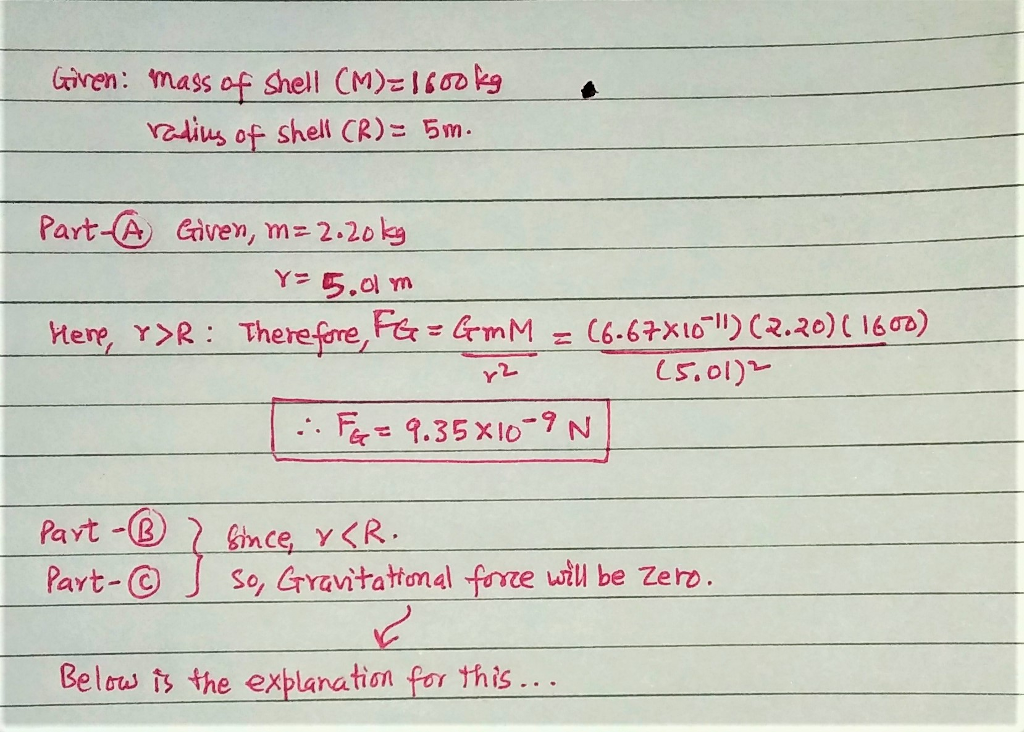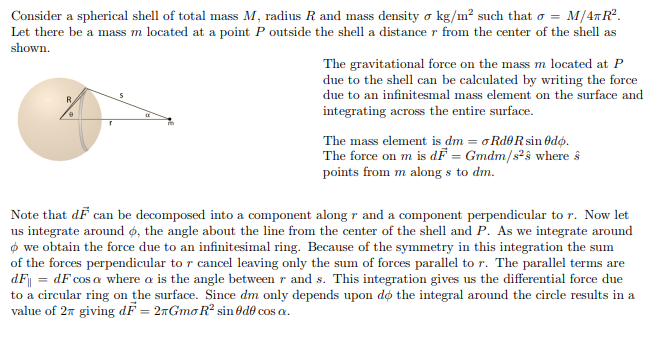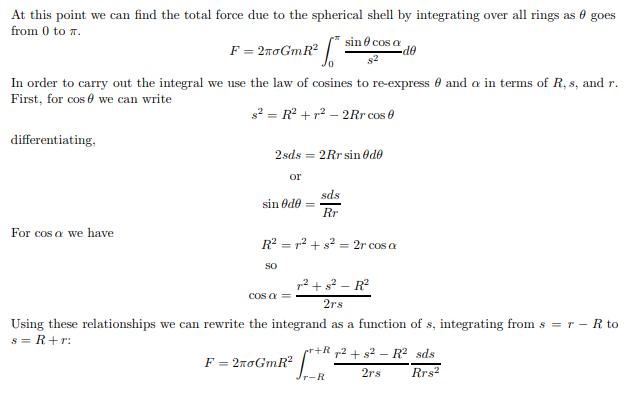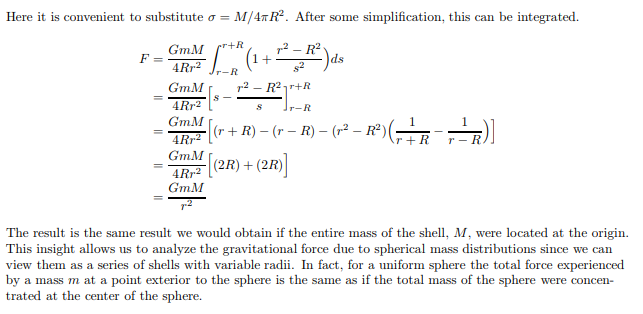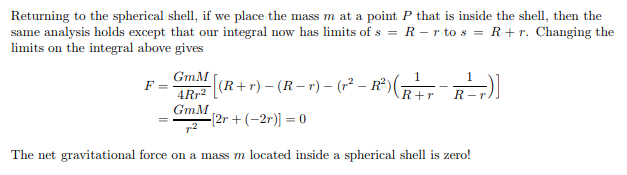> Thank you. A very clear and detailed explanation!

PhysicsStudent1 Tue, Nov 23, 2021 6:56 AM

#### Earn Coin

Coins can be redeemed for fabulous gifts.

Similar Homework Help Questions
• ### A uniform, solid, 1000.0 kg sphere has a radius of 5.00 m. Find the gravitational force...

A uniform, solid, 1000.0 kg sphere has a radius of 5.00 m. Find the gravitational force this sphere exerts on a 2.40 kg point mass placed 2.45 m from the center of the sphere

• ### A uniform, solid, 1700.0 kg sphere has a radius of 5.00 m . Find the gravitational...

A uniform, solid, 1700.0 kg sphere has a radius of 5.00 m . Find the gravitational force this sphere exerts on a 1.60 kg point mass placed at the following distances from the center of the sphere: (a) 5.02 m , and (b) 2.55 m .

• ### (a) A 1.06 kg mass is at the center of a uniform spherical shell of mass...

(a) A 1.06 kg mass is at the center of a uniform spherical shell of mass 1.10 1020 kg and radius 1.01 106 m. Find the resultant gravitational force on the mass. (b) Find the resultant gravitational force on the mass if it is placed just outside the shell. magnitude = (c) Find the value of g just outside the shell.

• ### Exercise 13.31 Constants Part A A uniform, solid, 1300.0 kg sphere has a radius of 5.00...Exercise 13.31 Constants Part A A uniform, solid, 1300.0 kg sphere has a radius of 5.00 m, Find the gravitational force this sphere exerts on a 1.60 kg point mass placed at the following distances from the center of the sphere: (a) 5.05 m, and (b) 2.45 m Submit Request Answer ▼ Part B A2p Submit Request Answer

• ### Exercise 13.31 Constants Part A A uniform, solid, 1300.0 kg sphere has a radius of 5.00...Exercise 13.31 Constants Part A A uniform, solid, 1300.0 kg sphere has a radius of 5.00 m, Find the gravitational force this sphere exerts on a 1.60 kg point mass placed at the following distances from the center of the sphere: (a) 5.05 m, and (b) 2.45 m Submit Request Answer ▼ Part B A2p Submit Request Answer

• ### A uniform, solid, 2000.0 kg sphere has a radius of 5.00 m. Find the gravitational force...

A uniform, solid, 2000.0 kg sphere has a radius of 5.00 m. Find the gravitational force this sphere exerts on a 1.80 kgpoint mass placed at the following distances from the center of the sphere: (a) 5.05 m , and (b) 2.30 m .

• ### Exercise 13.31 10 of 10 Constants A uniform, solid, 1500.0 kg sphere has a radius of...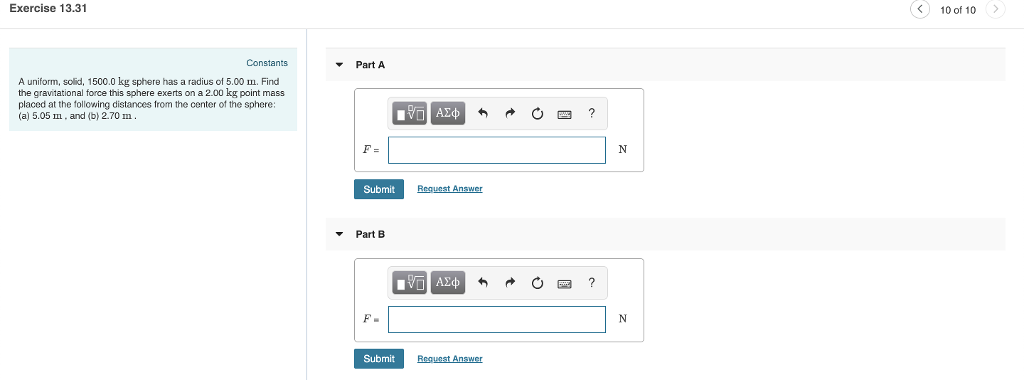Exercise 13.31 10 of 10 Constants A uniform, solid, 1500.0 kg sphere has a radius of 5.00 I. Find the gravitational force this sphere exerts on a 2.00 kg point mass placed at the following distances from the center of the sphere: (a) 5.05 m, and (b) 2.70 m Submit Part B F. Submit

• ### uniform solid sphere

A uniform solid sphere of mass m2 = 221 kg and radius R2 = 1.18 m is inside and concentric with a spherical shell of mass m1 = 165 kg and radius R1 = 2.44 m.a) Find the magnitude of the gravitational force exerted by the sphere and spherical shell on a particle of mass 5 kg located at a distance 0.64 m from the center ofthe sphere and spherical shell.b) Find the magnitude of the gravitational force exerted by...

• ### A thin metallic spherical shell of radius 0.387 m has a total charge of 9.03 ×...

A thin metallic spherical shell of radius 0.387 m has a total charge of 9.03 × 10-6 C placed on it. At the center of the shell is placed a point charge of 1.65 × 10-6 C. What is the electric field at a distance of 0.995 m from the center of the spherical shell?Express your answer in N/C

• ### 6) A thin metallic spherical shell of radius 0.177 m has a total charge of 9.03...

6) A thin metallic spherical shell of radius 0.177 m has a total charge of 9.03 × 10-6 C placed on it. At the center of the shell is placed a point charge of 2.65 × 10-6 C. What is the electric field at a distance of 0.815 m from the center of the spherical shell?